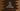# C++ program to find the power of a number# Introduction :

In this tutorial, we will learn how to find the power of a number in C++. We can use one loop to find the power or C++ provides one method called pow() to find the power.

The program will ask the user to enter two numbers: the base number and the exponent. It will calculate and print out the power using the base and exponent.

## Calculate power using a loop :

We can use any loop to solve it. In the program below, I am using one while loop :

``````#include <iostream>
using namespace std;

int main()
{
//1
float b, e, result = 1;

//2
cout << "Enter base : "; cin >> b;

cout << "Enter exponent : "; cin >> e;

//3
while (e != 0)
{
result *= b;
e--;
}

//4
cout << "Result : " << result << endl;
}
``````

### Explanation :

The commented numbers in the above program denote the step numbers below :

1. Create three floating-point numbers to store the user-provided values and the result values.

2. Ask the user to enter the base value. Read and store it in variable b. Similarly, read and store the exponent value in variable e.

3. Run one while loop to find out the final power i.e. b to the power e. For that, we need to multiply b to its own value e times. The while loop makes sure that the multiplication is done properly. It decrements the value of e on each iteration of the loop and stops the loop once the value of e reaches 0. Inside the loop, we are multiplying the value of b to result i.e. once the loop will end, result will hold the final power.

4. Finally, print out the result to the user.

### Sample output :

``````Enter base : 4
Enter exponent : 3
Result : 64
``````

Similarly, we can use one for loop or do while loop to find out the power of a number.

## Calculate power using pow() :

C++ header cmath includes one function called pow() to calculate the power of a number easily without writing any extra code. It is defined as below :

``````double pow(double base,double exponent)
``````

It takes two double parameters i.e. base, exponent and returns the power in double format.

``````#include <iostream>
#include <cmath>

using namespace std;

int main()
{
float b, e, result = 1;

cout << "Enter base : "; cin >> b;

cout << "Enter exponent : "; cin >> e;

result = pow(b,e);

cout << "Result : " << result << endl;
}
``````

The only thing you need to remember is that cmath is required to use pow().

### Conclusion :

In this tutorial, we have learned how to find the power of a number in C++ using two different approaches. You can write your own function to calculate the power or you can directly use the cmath header file for that. Try to go through the examples above and drop one comment below if you have any queries.